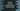# How to truncate datetime object and get the date in Python## How to truncate datetime object and get the date in Python:

datetime object holds both date and time values. But, sometimes we need to show only the date without any time values hour, minute, seconds.

In this post, we will learn how to truncate the time from a datetime object in Python with example.

## Method 1: By using date():

date() method of datetime module returns only the date component of datetime, excluding time part. We can use this method to print the date of a datetime.

``````from datetime import datetime

now = datetime.now()

print(now.date())``````

It will print something like below:

``2020-08-18``

## Method 2: By using strftime():

strftime takes one string format and it formats the datetime object based on that. We can pass one string that includes only day, month and year to get the required result:

``````from datetime import datetime

now = datetime.now()

print(now.strftime("%d/%m/%Y"))``````

It will print:

``18/08/2020``

The advantage of this method is that we can change the format of the output string to whatever we like.

Similarly, if you want the month in words, you can do something as like below:

``````from datetime import datetime

now = datetime.now()

print(now.strftime("%d/%b/%Y"))``````

It will print:

``18/Aug/2020``

or if you want the month in full:

``````from datetime import datetime

now = datetime.now()

print(now.strftime("%d/%B/%Y"))``````

It will give:

``18/August/2020``

## Method 3: By reading the properties:

datetime object has day, month and year properties. We can read these properties to create a date string with day, month and year.

For example,

``````from datetime import datetime

now = datetime.now()

print(f'{now.day}::{now.month}::{now.year}')``````

It will print something like below:

``18::8::2020``